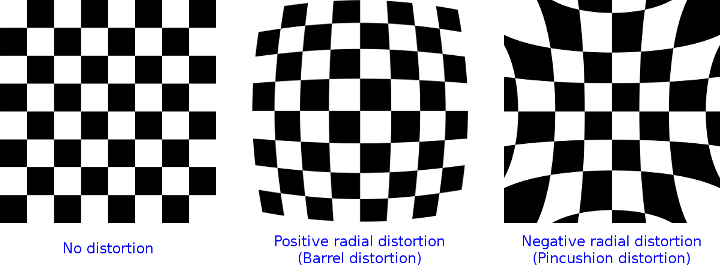# Are Lens Distortion Coefficients inverted for projectPoints?

Good day guys,

I am trying to simulate and image with lens barrel distortion. I create a virtual chessboard (only the corners) and then project it onto my image plane using OpenCV. The idea is to project these points with known distortion coefficients, and then attempt a lens distortion calibration (with calibrateCamera), and see if the same coefficients are obtained.

My question is about the projectPoints function which takes distCoeffs as an input. Are these coefficients the same that must be used to undistort an image (output of calibrateCamera)? This means the function will have to calculate the inverse of that operation. Or, does it use those coefficients to distort the object points directly? Meaning that the will not correlate at all at the output of e.g. calibrateCamera.

I ask, because I noticed my simulation does pincushion distortion when I expect barrel, and vica versa. Which seems that the distortion does the opposite of what I think it does.

The minimal working code that I used to simulate the image (in Python):

# The distortion matrix that I vary
distortion = np.array([0.03, 0.0001, 0.0, 0.0, 0.0001])

# Generate Grid of Object Points
grid_size, square_size = [20, 20], 0.2
object_points = np.zeros([grid_size * grid_size, 3])
mx, my = [(grid_size - 1) * square_size / 2, (grid_size - 1) * square_size / 2]
for i in range(grid_size):
for j in range(grid_size):
object_points[i * grid_size + j] = [i * square_size - mx, j * square_size - my, 0]

# Setup the camera information
f, p = [5e-3, 120e-8]
intrinsic = np.array([[f/p, 0, 0], [0, f/p, 0], [0, 0, 1]])
rvec = np.array([0.0, 0.0, 0.0])
tvec = np.array([0.0, 0.0, 3.0])

# Project the points
image_points, jacobian = cv2.projectPoints(object_points, rvec, tvec, intrinsic, distortion)

# Plot the points (using PyPlot)
plt.scatter(*zip(*image_points[:, 0, :]), marker='.')
plt.axis('equal')
plt.xlim((-4000, 4000))
plt.ylim((-4000, 4000))
plt.grid()
plt.show()


edit retag close merge delete

Sort by » oldest newest most voted

with k1 > 0with k1<0Following the wiki the effect should be the opposite as @Stringweasel pointed out

...typically k1>0 and pincushion distortion (typically k1<0)...The vectors I used are, respectively,

D_00 = np.array([ 0.25, 0.0, 0.0, 0.0, 0.0], dtype=np.float64)
D_00 = np.array([ -0.25, 0.0, 0.0, 0.0, 0.0], dtype=np.float64)


Does anyone have a clue about what's going on?

more

1

i have just created an extended and more detailed version of the both question and "my answer" (that is not an answer...) here https://answers.opencv.org/question/2...

After thinking about this issue, i think they're not really "inverted" , it's a chicken-and-egg problem.. it really depends on how we imagine and try to reconstruct the "physics" :-)

Official site

GitHub

Wiki

Documentation